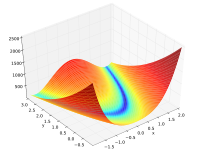### Summary

Kernel methods are a family of methods that involve a function $k(x,y)$ that describes the expected similarity of function values at points $x$ and $y$. These methods are sometimes interpreted probabilistically, with the kernel representing the covariance of random variables at different locations, and sometimes they are interpreted deterministically. These different interpretations provide distinct ways of thinking about kernel selection and error analysis. Independent of the interpretation, kernel methods tend to result in large, dense, highly-structured linear matrix problems; computations with these matrices are a serious bottleneck in scaling to large data sets. In this line of work, we consider both the theoretical aspects of kernel-based function approximation schemes and the nuts-and-bolts of algorithms that exploit matrix structure in order to scale to large data sets.

### Related#### Parallel surrogate optimization

Asynchronous parallel algorithms for finding minima fast by fitting functions to surrogate models.

### Papers

D. Eriksson, K. Dong, E. Lee, D. Bindel, and A. G. Wilson, “Scaling Gaussian Process Regression with Derivatives,” in Proceedings of NeurIPS 2018, 2018.
@inproceedings{2018-nips-a,
author = {Eriksson, David and Dong, Kun and Lee, Eric and Bindel, David and Wilson, Andrew Gordon},
title = {Scaling {Gaussian} Process Regression with Derivatives},
booktitle = {Proceedings of NeurIPS 2018},
month = dec,
year = {2018},
arxiv = {1810.12283.pdf},
}


#### Abstract:

Gaussian processes (GPs) with derivatives are useful in many applications, including Bayesian optimization, implicit surface reconstruction, and terrain reconstruction. Fitting a GP to function values and derivatives at $n$ points in $d$ dimensions requires linear solves and log determinants with an $n(d + 1) × n(d + 1)$ positive definite matrix – leading to prohibitive $O(n^3 d^3)$ computations for standard direct methods. We propose iterative solvers using fast $O(nd)$ matrix-vector multiplications (MVMs), together with pivoted Cholesky preconditioning that cuts the iterations to convergence by several orders of magnitude, allowing for fast kernel learning and prediction. Our approaches, together with dimensionality reduction, enables Bayesian optimization with derivatives to scale to high-dimensional problems and large evaluation budgets.

J. Gardner, G. Pleiss, K. Weinberger, D. Bindel, and A. G. Wilson, “GPyTorch: Blackbox Matrix-Matrix Gaussian Process Inference with GPU Acceleration,” in Proceedings of NeuroIPS 2018, 2018.
@inproceedings{2018-nips-b,
author = {Gardner, Jacob and Pleiss, Geoff and Weinberger, Killian and Bindel, David and Wilson, Andrew Gordon},
title = {{GPyTorch}: Blackbox Matrix-Matrix {Gaussian} Process Inference with {GPU} Acceleration},
booktitle = {Proceedings of NeuroIPS 2018},
month = dec,
year = {2018},
arxiv = {1809.11165.pdf},
}


#### Abstract:

Despite advances in scalable models, the inference tools used for Gaussian processes (GPs) have yet to fully capitalize on developments in computing hardware. We present an efficient and general approach to GP inference based on Blackbox Matrix-Matrix multiplication (BBMM). BBMM inference uses a modified batched version of the conjugate gradients algorithm to derive all terms for training and inference in a single call. BBMM reduces the asymptotic complexity of exact GP inference from $O(n^3)$ to $O(n^2)$. Adapting this algorithm to scalable approximations and complex GP models simply requires a routine for efficient matrix-matrix multiplication with the kernel and its derivative. In addition, BBMM uses a specialized preconditioner to substantially speed up convergence. In experiments we show that BBMM effectively uses GPU hardware to dramatically accelerate both exact GP inference and scalable approximations. Additionally, we provide GPyTorch, a software platform for scalable GP inference via BBMM, built on PyTorch.

K. Dong, D. Eriksson, H. Nickisch, D. Bindel, and A. G. Wilson, “Scalable Log Determinants for Gaussian Process Kernel Learning,” in Proceedings of NIPS 2017, 2017.
@inproceedings{2017-nips,
author = {Dong, Kun and Eriksson, David and Nickisch, Hannes and Bindel, David and Wilson, Andrew Gordon},
booktitle = {Proceedings of NIPS 2017},
title = {Scalable Log Determinants for Gaussian Process Kernel Learning},
year = {2017},
month = dec,
arxiv = {1711.03481.pdf}
}


#### Abstract:

For applications as varied as Bayesian neural networks, determinantal point processes, elliptical graphical models, and kernel learning for Gaussian processes (GPs), one must compute a log determinant of an $n \times n$ positive definite matrix, and its derivatives – leading to prohibitive $O(n^3)$ computations. We propose novel $O(n)$ approaches to estimating these quantities from only fast matrix vector multiplications (MVMs). These stochastic approximations are based on Chebyshev, Lanczos, and surrogate models, and converge quickly even for kernel matrices that have challenging spectra. We leverage these approximations to develop a scalable Gaussian process approach to kernel learning. We find that Lanczos is generally superior to Chebyshev for kernel learning, and that a surrogate approach can be highly efficient and accurate with popular kernels.

### Talks

#### Stochastic Linear Algebra for Scalable Gaussian Processes

Applied Mathematics Colloquium, Illinois Institute of Technology
gp kernelcolloquium external invited

#### Stochastic Linear Algebra for Scalable Gaussian Processes

CAM Colloquium, University of Chicago
gp kernelcolloquium external invited

#### Stochastic Linear Algebra for Scalable Gaussian Processes

IEMS Seminar, Northwestern University
gp kernelseminar external invited

#### LA Support for Scalable Kernel Methods

Workshop on Approximation Theory and Machine Learning, Purdue
gp kernelmeeting external invited

#### Stochastic LA for Scaling GPs

International Workshop on Computational Math, Suzhou
gp kernelmeeting external invited

#### Scalable Algorithms for Kernel-Based Surrogates in Prediction and Optimization

Workshop on Surrogate Models and Coarsening Techniques, UCLA IPAM
gp kernelmeeting external invited

#### Stochastic LA for Scaling GPs

Cornell SCAN seminar
gp kernelseminar local

#### Stochastic estimators in Gaussian process kernel learning

Householder Symposium
gp kernelmeeting external invited poster

#### RBF Response Surfaces with Inequality Constraints

SIAM CSE
rbfminisymposium external organizer

#### Radial Basis Function Interpolation with Bound Constraints

Cornell SCAN Seminar
rbfseminar local